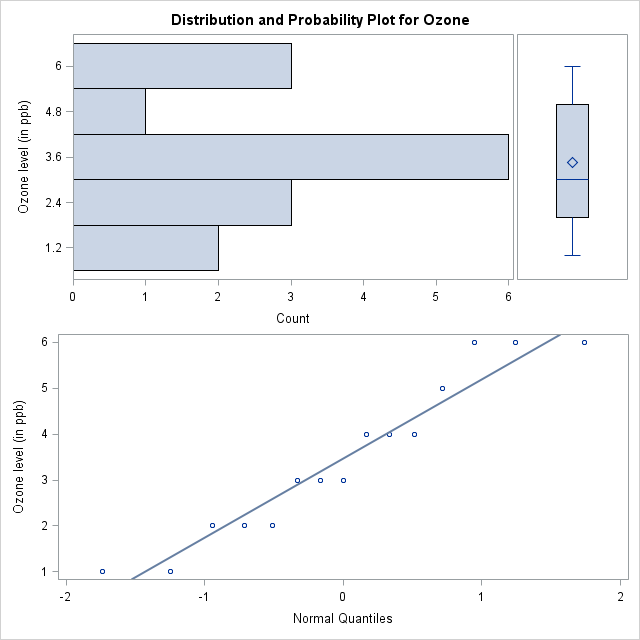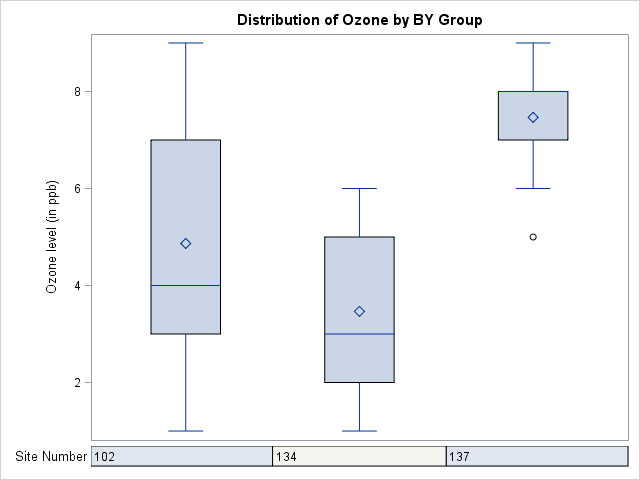# The UNIVARIATE Procedure

### Example 4.5 Creating Basic Summary Plots

The PLOTS option in the PROC UNIVARIATE statement requests several basic summary plots. For more information about plots created by the PLOTS option, see the section Creating Line Printer Plots. This example illustrates the use of the PLOT option as well as BY processing in PROC UNIVARIATE.

A researcher is analyzing a data set consisting of air pollution data from three different measurement sites. The data set `AirPoll`, created by the following statements, contains the variables `Site` and `Ozone`, which are the site number and ozone level, respectively.

```data AirPoll (keep = Site Ozone);
label Site  = 'Site Number'
Ozone = 'Ozone level (in ppb)';
do i = 1 to 3;
input Site @@;
do j = 1 to 15;
input Ozone @@;
output;
end;
end;
datalines;
102 4 6 3 4 7 8 2 3 4 1 3 8 9 5 6
134 5 3 6 2 1 2 4 3 2 4 6 4 6 3 1
137 8 9 7 8 6 7 6 7 9 8 9 8 7 8 5
;
```

The following statements produce basic plots for each site in the `AirPoll` data set:

```ods graphics off;
ods select Plots SSPlots;
proc univariate data=AirPoll plot;
by Site;
var Ozone;
run;
```

The ODS GRAPHICS OFF statement specified before the PROC statement disables ODS Graphics, which causes the PLOTS option to produce legacy line printer plots. The PLOTS option produces a stem-and-leaf plot, a box plot, and a normal probability plot for the `Ozone` variable at each site. Because a BY statement is specified, a side-by-side box plot is also created to compare the ozone levels across sites. Note that `AirPoll` is sorted by `Site`; in general, the data set should be sorted by the BY variable by using the SORT procedure. The ODS SELECT statement restricts the output to the "Plots" and "SSPlots" tables; see the section ODS Table Names. Optionally, you can specify the PLOTSIZE=n option to control the approximate number of rows (between 8 and the page size) that the plots occupy.

Output 4.5.1 through Output 4.5.3 show the plots produced for each BY group. Output 4.5.4 shows the side-by-side box plot for comparing `Ozone` values across sites.

Output 4.5.1: Ozone Plots for BY Group Site = 102

 Analysis of Score Changes

The UNIVARIATE Procedure
Variable: Ozone (Ozone level (in ppb))

 ``` Stem Leaf # Boxplot 9 0 1 | 8 00 2 | 7 0 1 +-----+ 6 00 2 | | 5 0 1 | | 4 000 3 *--+--* 3 000 3 +-----+ 2 0 1 | 1 0 1 | ----+----+----+----+ Normal Probability Plot 9.5+ *++++ | * * ++++ | * +++++ | * *+++ 5.5+ +*++ | **+* | * *+*+ | *++++ 1.5+ *++++ +----+----+----+----+----+----+----+----+----+----+ -2 -1 0 +1 +2 ```

Output 4.5.2: Ozone Plots for BY Group Site = 134

 Analysis of Score Changes

The UNIVARIATE Procedure
Variable: Ozone (Ozone level (in ppb))

 ``` Stem Leaf # Boxplot 6 000 3 | 5 0 1 +-----+ 4 000 3 | | 3 000 3 *--+--* 2 000 3 +-----+ 1 00 2 | ----+----+----+----+ Normal Probability Plot 6.5+ * * ++*+++ | * ++++++ | **+*+++ | **+*+++ | *+*+*++ 1.5+ * ++*+++ +----+----+----+----+----+----+----+----+----+----+ -2 -1 0 +1 +2 ```

Output 4.5.3: Ozone Plots for BY Group Site = 137

 Analysis of Score Changes

The UNIVARIATE Procedure
Variable: Ozone (Ozone level (in ppb))

 ``` Stem Leaf # Boxplot 9 000 3 | 8 | 8 00000 5 +-----+ 7 | + | 7 0000 4 +-----+ 6 | 6 00 2 | 5 5 0 1 0 ----+----+----+----+ Normal Probability Plot 9.25+ * *++++* | ++++ | * ** *+*+ | ++++ 7.25+ * * **++ | +++++ | *++* | ++++ 5.25+ +++* +----+----+----+----+----+----+----+----+----+----+ -2 -1 0 +1 +2 ```

Output 4.5.4: Ozone Side-by-Side Boxplot for All BY Groups

 Analysis of Score Changes

The UNIVARIATE Procedure
Variable: Ozone (Ozone level (in ppb))

Schematic Plots

 ``` | 9 + | | | | | | | | | | | 8 + | *-----* | | | | | | | + | | | | | 7 + +-----+ +-----+ | | | | | | | | | | | | 6 + | | | | | | | | | | | | | | | | 5 + | | +-----+ 0 | | + | | | | | | | | | | | | | 4 + *-----* | | | | | | | | | | | + | | | | | | 3 + +-----+ *-----* | | | | | | | | | | | | 2 + | +-----+ | | | | | | | | | 1 + | | ------------+-----------+-----------+----------- Site 102 134 137 ```

The following statements produce basic plots by using ODS Graphics:

```ods graphics on;
ods select Plots SSPlots;
proc univariate data=AirPoll plot;
by Site;
var Ozone;
run;
```

Output 4.5.5 through Output 4.5.8 show the plots produced by using ODS Graphics. Note that the line printer stem-and-leaf plots are replaced by horizontal histograms in ODS Graphics output.

Output 4.5.5: Ozone Plots for BY Group Site = 102Output 4.5.6: Ozone Plots for BY Group Site = 134Output 4.5.7: Ozone Plots for BY Group Site = 137Output 4.5.8: Ozone Side-by-Side Boxplot for All BY GroupsNote: You can use the PROBPLOT statement with the NORMAL option to produce high-resolution normal probability plots; see the section Modeling a Data Distribution. You can use the BOXPLOT procedure to produce box plots that use high-resolution graphics. See Chapter 28: The BOXPLOT Procedure in SAS/STAT 14.2 User's Guide.

A sample program for this example, uniex04.sas, is available in the SAS Sample Library for Base SAS software.